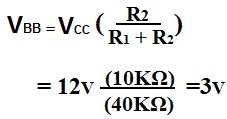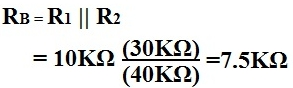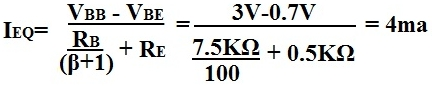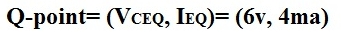﻿ How to Find the Q-point of a Transistor Circuit# How to Find the Q-point of a Transistor Circuit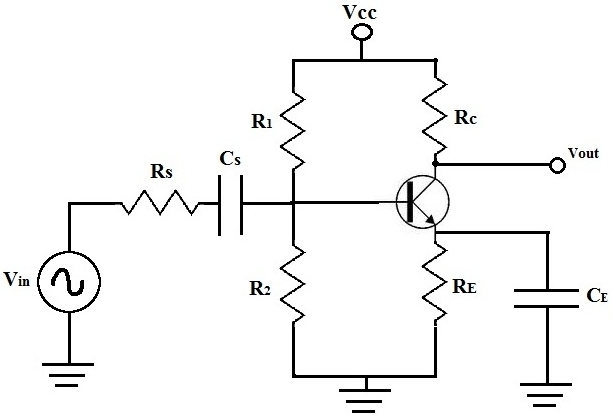What is shown above is a typical transistor circuit.

In this article, we're going to show how to find the quiescient or just simply the q-point of a Transistor Circuit.

In order to do this, all we have to do is DC analysis of the transistor circuit. From that alone, we can find its q-point.

When doing DC analysis, all AC voltage sources are taken out of the circuit because they're AC sources. DC analysis is concerned only with DC sources. We also take out all capacitors because in DC, capacitors are open. For this reason, everything before and after capacitors are removed, which in this circuit includes resistor, Rs.

Below is the schematic of the circuit above with respective to DC analysis: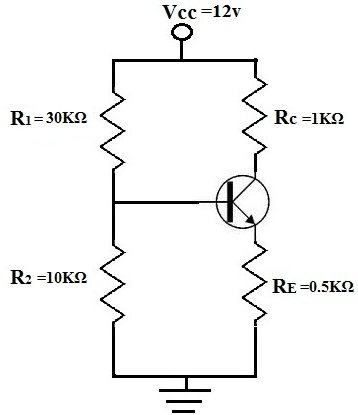Now let's do the calculations to find the Vbb, Rb, Ieq, and Vceq. From this then, we can find the q-point of this transistor circuit.